# Star Delta Starter: Circuit Diagram, Working Principle & Theory

## What is Star Delta Starter

A star delta starter is the most commonly used method for the starting of a 3 phase induction motor. In star delta starting an induction motor is connected in through a star connection throughout the starting period. Then once the motor reaches the required speed, the motor is connected in through a delta connection.

A star delta starter will start a motor with a star connected stator winding. When motor reaches about 80% of its full load speed, it will begin to run in a delta connected stator winding.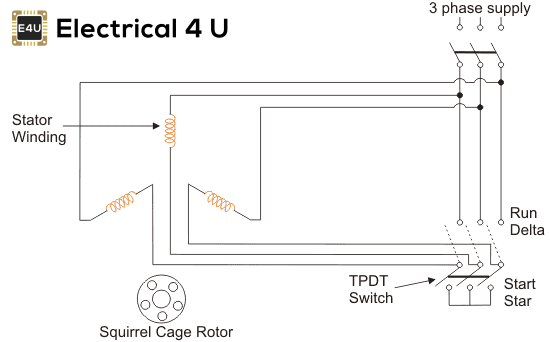A star delta starter is a type of reduced voltage starter. We use it to reduce the starting current of the motor without using any external device or apparatus. This is a big advantage of a star delta starter, as it typically has around 1/3 of the inrush current compared to a DOL starter.

The starter mainly consists of a TPDP switch which stands for Tripple Pole Double Throw switch. This switch changes stator winding from star to delta. During starting condition stator winding is connected in the form of a star. Now we shall see how a star delta starter reduces the starting current of a three-phase induction motor.

For that let us consider,

VL = Supply Line Voltage, ILS = Supply Line Current and, IPS = Winding Current per Phase and Z = Impedance per phase winding at stand still condition.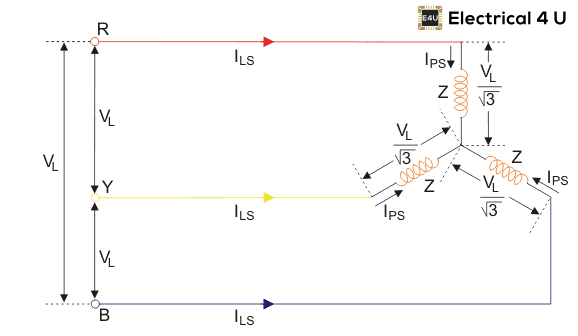As the winding is star connected, the winding current per phase (IPS) equals to supply line current (ILS).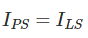As the winding is star connected, the voltage across each phase of the winding is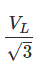Hence, the winding current per phase is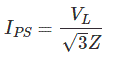Since here, the winding current per phase (IPS) equals to the supply line current (ILS), we can write,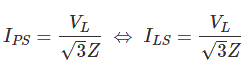Now, let us consider the situation where the motor gets started with delta connected stator winding from same three phase supply points,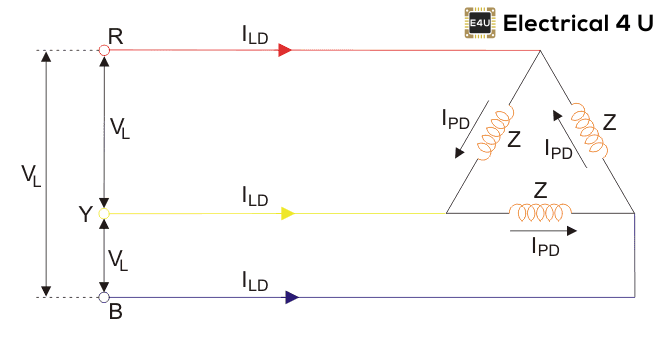Here, ILD = Supply Line Current and, IPD = Winding Current per Phase and Z = Impedance per phase winding at stand still condition.
As the winding is delta connected, supply line current (ILD) is root three times of the winding current per phase (IPD)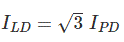As the winding is delta connected, the voltage across each phase of the winding is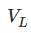Hence, the winding current per phase is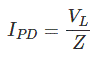Now, we can write,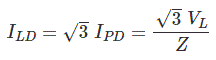Now, by comparing supply line currents drawn by an induction motor with star and delta connected winding, we get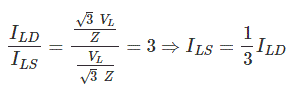Thus we can say that the starting current from the mains in case of star delta is one-third of direct switching in the delta. Again, we know that the starting torque of an induction motor is proportional to the square of the voltage applied to the winding per phase.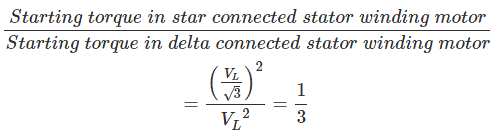The equation shows that star delta starter reduces the starting torque to one-third of that produced by DOL starter. The star-delta starter is equivalent to an autotransformer with a 57.7% tapping.

## Advantages of Star Delta Starter

The advantages of star delta starters include:

1. Inexpensive
2. No heat is produced, or tap changing device needs to be used, hence efficiency increases.
3. Starting current reduced to 1/3 of direct online starting current.
4. Produce high torque per ampere of line current.

## Disadvantages of Star Delta Starter

The disadvantages of star delta starters include:

1. Starting torque is reduced to 1/3 of full load torque.
2. A particular set of motors required.

## Application of Star Delta Starter

As discussed in the above advantages and disadvantages, a star delta starter is most suited to applications where the required starting current is low and where the line current draw must be at a minimum value.

The star delta starter is not suitable for applications where high starting torque delivery is required. For these applications, a DOL starter should be used instead.

If the motor is too heavily loaded, there will not be enough torque to accelerate the motor upto speed before switching over to the delta position. Example application for a star delta starter is a Centrifugal compressor.

Want To Learn Faster? 🎓
Get electrical articles delivered to your inbox every week.
No credit card required—it’s 100% free.